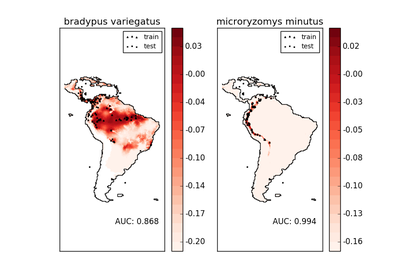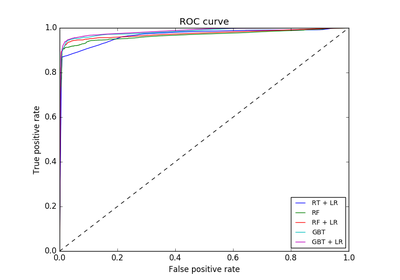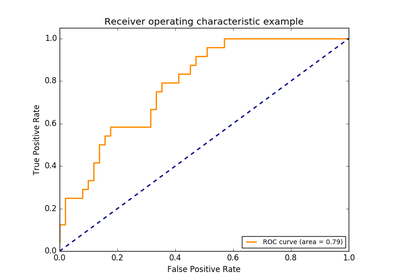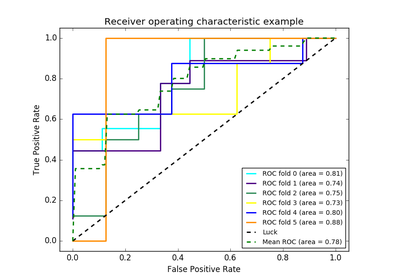# `sklearn.metrics`.roc_curve¶

`sklearn.metrics.``roc_curve`(y_true, y_score, pos_label=None, sample_weight=None, drop_intermediate=True)[source]

Note: this implementation is restricted to the binary classification task.

Read more in the User Guide.

Parameters: y_true : array, shape = [n_samples] True binary labels in range {0, 1} or {-1, 1}. If labels are not binary, pos_label should be explicitly given. y_score : array, shape = [n_samples] Target scores, can either be probability estimates of the positive class, confidence values, or non-thresholded measure of decisions (as returned by “decision_function” on some classifiers). pos_label : int or str, default=None Label considered as positive and others are considered negative. sample_weight : array-like of shape = [n_samples], optional Sample weights. drop_intermediate : boolean, optional (default=True) Whether to drop some suboptimal thresholds which would not appear on a plotted ROC curve. This is useful in order to create lighter ROC curves. New in version 0.17: parameter drop_intermediate. fpr : array, shape = [>2] Increasing false positive rates such that element i is the false positive rate of predictions with score >= thresholds[i]. tpr : array, shape = [>2] Increasing true positive rates such that element i is the true positive rate of predictions with score >= thresholds[i]. thresholds : array, shape = [n_thresholds] Decreasing thresholds on the decision function used to compute fpr and tpr. thresholds represents no instances being predicted and is arbitrarily set to max(y_score) + 1.

`roc_auc_score`
Compute Area Under the Curve (AUC) from prediction scores

Notes

Since the thresholds are sorted from low to high values, they are reversed upon returning them to ensure they correspond to both `fpr` and `tpr`, which are sorted in reversed order during their calculation.

References

Examples

```>>> import numpy as np
>>> from sklearn import metrics
>>> y = np.array([1, 1, 2, 2])
>>> scores = np.array([0.1, 0.4, 0.35, 0.8])
>>> fpr, tpr, thresholds = metrics.roc_curve(y, scores, pos_label=2)
>>> fpr
array([ 0. ,  0.5,  0.5,  1. ])
>>> tpr
array([ 0.5,  0.5,  1. ,  1. ])
>>> thresholds
array([ 0.8 ,  0.4 ,  0.35,  0.1 ])
```

## Examples using `sklearn.metrics.roc_curve`¶Species distribution modelingFeature transformations with ensembles of trees